Courses

# Test: Problems On Similar Triangles

## 10 Questions MCQ Test Mathematics (Maths) Class 10 | Test: Problems On Similar Triangles

Description
This mock test of Test: Problems On Similar Triangles for Class 10 helps you for every Class 10 entrance exam. This contains 10 Multiple Choice Questions for Class 10 Test: Problems On Similar Triangles (mcq) to study with solutions a complete question bank. The solved questions answers in this Test: Problems On Similar Triangles quiz give you a good mix of easy questions and tough questions. Class 10 students definitely take this Test: Problems On Similar Triangles exercise for a better result in the exam. You can find other Test: Problems On Similar Triangles extra questions, long questions & short questions for Class 10 on EduRev as well by searching above.
QUESTION: 1

### Consider the triangle shown in the figure where BC = 12 cm, Db = 9 cm, CD = 6 cm and What is the ratio of the perimeter of the triangle ADC to that of the triangle BDC?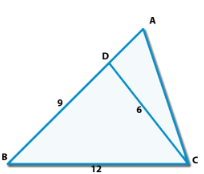Solution:

Here, ∠ACB = c+180-(2c-b) = 180-(b+c)
So, We can say that Δ BCD and &delta ABC will be similar.
According to property of similarity,
AB/12 = 12/9
Hence,
AB = 16
AC/6 = 12/9
AC = 8
Hence, AD = 7 and AC = 8
Now,
Perimeter of Δ ADC / Perimeter of ΔBDC,
= (6+7+8)/(9+6+12)
= 21/27 = 7/9.

QUESTION: 2

Solution:
QUESTION: 3

### If ABC and PQR are similar triangles in which ∠ A = 470 and ∠ Q = 830, then ∠ C is:

Solution:
QUESTION: 4

In the given figure, T and B are right angles. If the lengths of AT, BC and AS (in centimeters) are 15, 16 and 17 respectively, then the length of TC (in centimeters) is: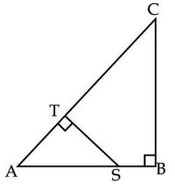Solution: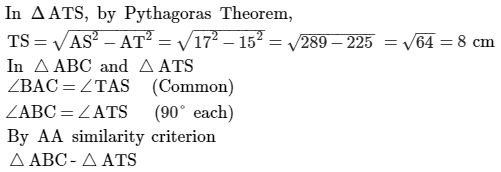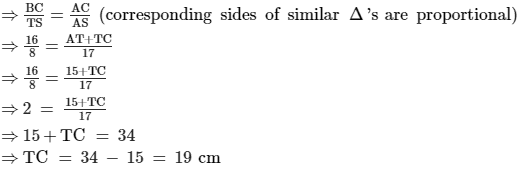QUESTION: 5

In the adjoining figure, AD = 2 cm, DB = 3 cm, AE = 5 cm and DE || BC, then find EC.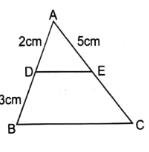Solution:
QUESTION: 6

In an equilateral triangle ABC, if AD ⊥ BC. Then​

Solution:

∆ ABC, in which sides are AB=BC= AC= a units and AD is perpendicular to BC ,

In ∆ADB ,

AB²= AD²+ BD²     (by Pythagoras theorem)

a² = AD² + (a/2)²   [BD= 1/2BC, since in an equilateral triangle altitude AD is  perpendicular bisector of BC ]

a²- a²/4 =AD²

⇒ ( 4a²-a²)/4 = AD²

⇒ 3a² /4 = AD²

⇒ 3AB²/4= AD²               [ AB= a]

⇒  3AB²= 4AD²

QUESTION: 7

In two triangles ABC and PQR,Given that ∠A = ∠R and ∠B = ∠Q, which of the following is true?​

Solution:

∠A = ∠R and ∠B = ∠Q
The names of triangles are written in such a manner in which the Angles are correspondingly equal which means that the vertices at which the angles of the two triangles are equal is placed accordingly. So since the the angle A and Angle R are equal and Angle B and Angle D are equal we have ΔABC - ΔRQP

QUESTION: 8

Triangles ABC, DEF are similar, ∠A = 75° , ∠B = 85° so ∠F = ?​

Solution:
QUESTION: 9

A vertical stick 30 m long casts a shadow 15 m long on the ground. At the same time, a tower casts a shadow 75 m long on the ground. The height of the tower is:​

Solution: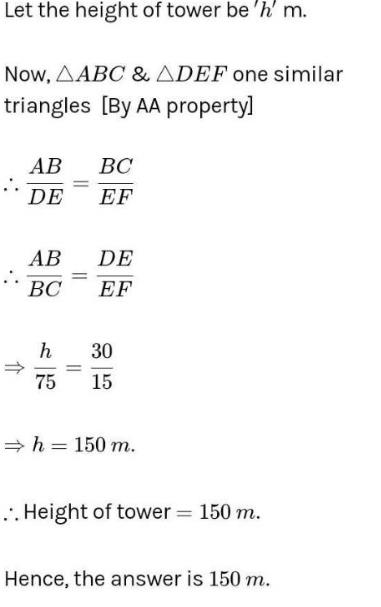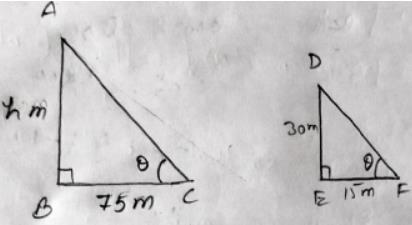QUESTION: 10

Given two triangles ABC and PQR such that, AB = 2 cm, PQ = 3cm, ∠B = ∠Q BC = 5 cm, QR = 7.5 cm. AG and PS are medians . Find AG/PS = ?​

Solution: